# Mechanomy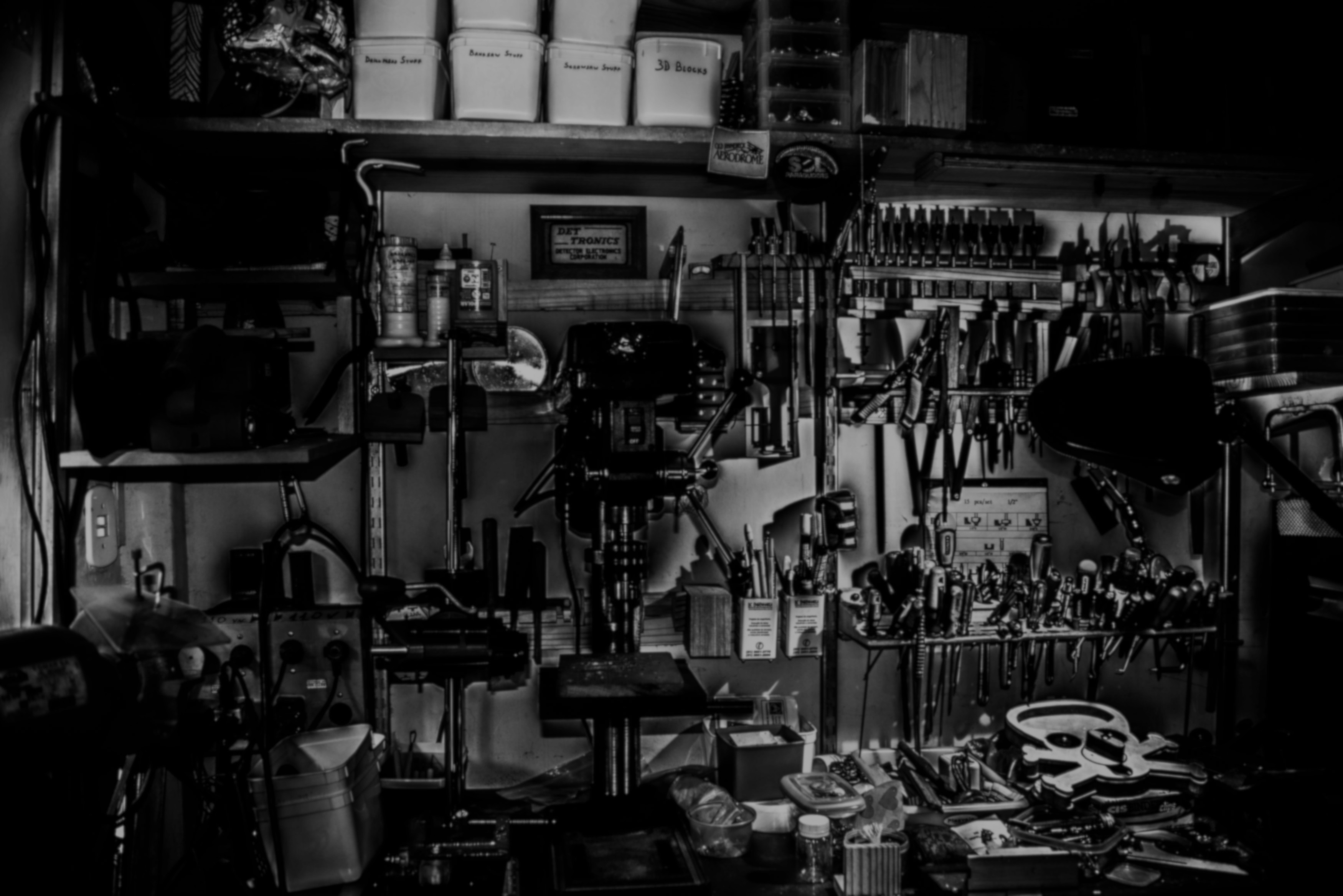# Sketching Involutes

Gears are foundational to many mechanisms, and it is the involute curve that is responsible for many of their capabilities. In this MonthlyMechanism we're calculating and sketching the involute curve.

Many references describe involute curves by analogy to a taut string unwrapping from a cylinder, and some depict a mechanical curve generator. But these physical approaches do not translate into CAD, often forcing designers to find a commercial gear for basic design work. This post derives the involute function from a circle, explains the geometry, presents a function to calculate a gear tooth involute, and compares this to a commercially-available gear.

## Deriving the involute of a circle #

Involutes are typically described as the points that a string traces when it is unwrapped from a circle or other curve. More useful is the property that every point on the involute is tangent to the involute and tangent to some ray of the generating circle. This can be seen graphically as: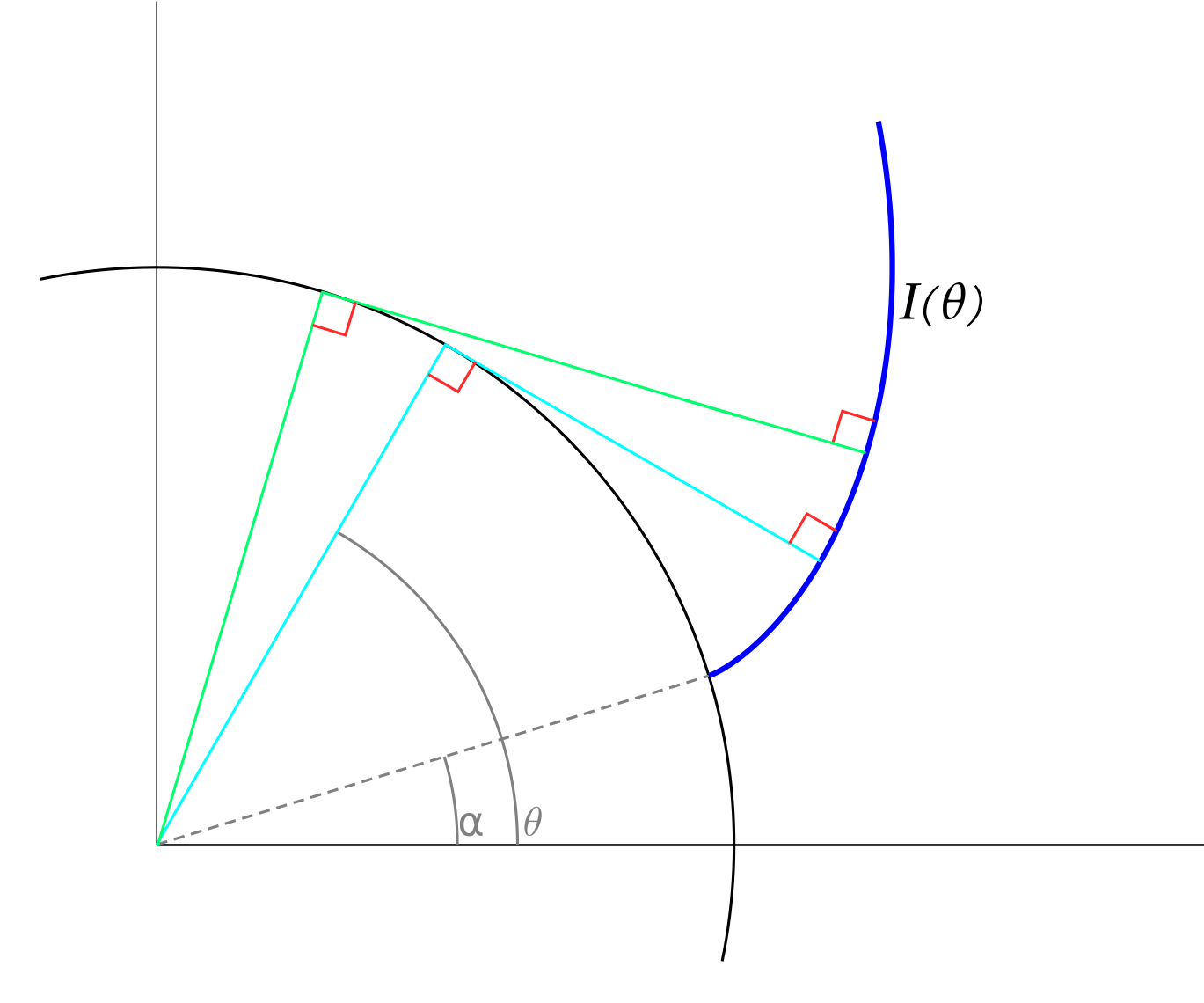As θ\thetaθ varies, points on the involute are mutually tangent to the circle.

Involutes are expressed mathematically as

$\vec{I}_{\alpha}(\theta) = \vec{c}(\theta) - { \frac{ \vec{c}^\prime(\theta)}{|\vec{c}^\prime (\theta)|}} \int _{\alpha}^{\theta} | \vec{c}^\prime(w)| dw$

with $\vec{c}(\theta)$ being a curve parameterized by $\theta$. In spur gearing the curve is a circle and $\vec{c}(\theta) = [ r\cos(\theta), r\sin(\theta) ]$.

Taking the derivative of $\vec{c}(\theta)$ with respect to $\theta$ gives

$\vec{c}^\prime(\theta)=[-r\sin\theta, r\cos\theta]$.

The magnitude of $\vec{c}^\prime(\theta)$ simplifies to $|\vec{c}^\prime(\theta)|=r$.

Putting those into the expression of the involute, integrating from $\alpha$ to $\theta$, and simplifying gives an equation for the involute of a circle in [x, y]:

$\vec{I}(\theta) = [ r\cos\theta + r(\theta-\alpha)\sin\theta, r\sin\theta - r(\theta-\alpha)\cos\theta ]$

Plotting this while varying $\theta$ traces the involute, while changing $\alpha$ moves the root of the involute.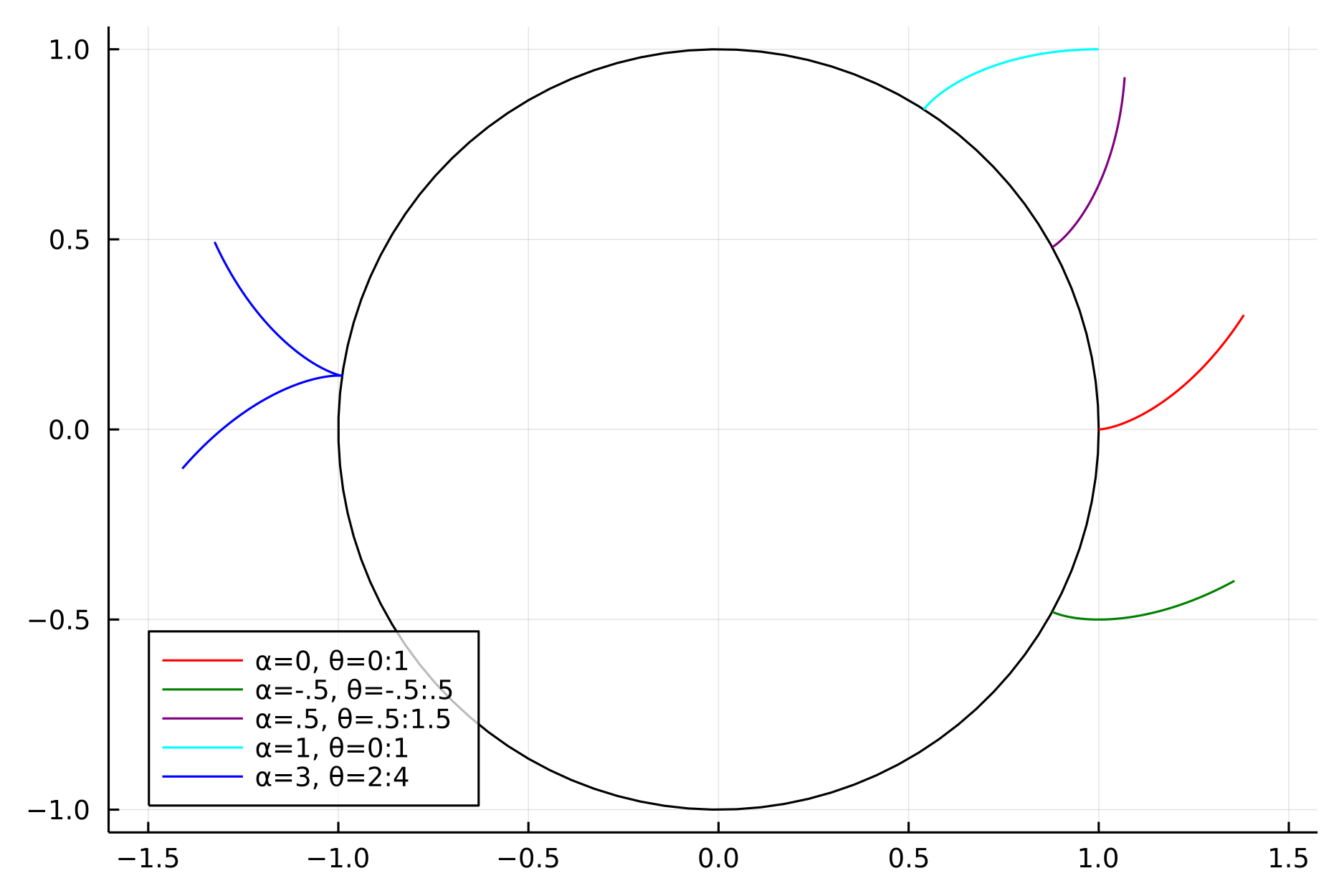Varying θ\thetaθ generates the involute curve, while α\alphaα positions the root of the curve around the gear.

## Sketching #

While the equation for an involute is essential, we need some geometry to apply it to gear teeth. Gear teeth are symmetric, often described as having a width and height, and arranged at regular angles.

Let $\gamma$ define the angle of the symmetric axis and thereby the overall angular location of the tooth about the gear, $\delta$ the angular width, and $r$ the radius of the circle at the involute's base. With these, the root angles of the two involutes that form the gear tooth are $\alpha = \gamma - \delta/2$ and $\beta = \gamma + \delta/2$:

The tooth will be formed from two involutes, one for each flank, and so we need to calculate the starting and stopping angles for each involute equation. The starting angles are $\alpha$ and $\beta$, but the stopping angles depend on the tooth height, as measured along the symmetry axis. Calculating a $\theta$ that leads to a particular tooth height requires a bit of geometry, here's the basic setup: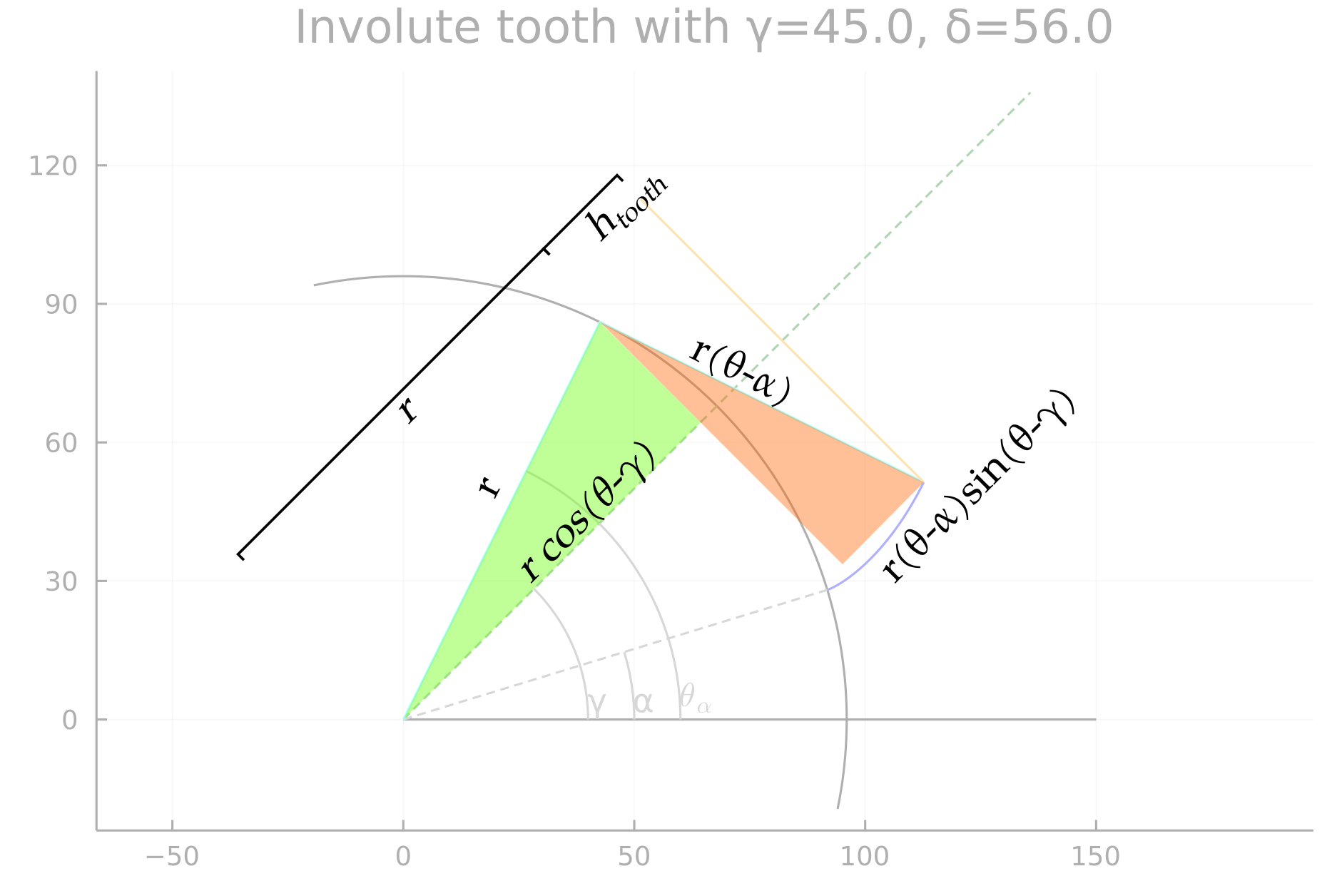We are trying to find θ\thetaθ, knowing rrr, γ\gammaγ, α\alphaα, and the desired tooth height htoothh_{tooth}htooth​. To do this we equate the radial distance of the top land of the tooth from the known rrr and htoothh_{tooth}htooth​ to the sum of the sides of the green and orange triangles.

The result is

$h_{tooth} = r ( \cos(\theta-\gamma) + (\theta-\alpha)\sin(\theta-\gamma) - 1 )$

for the flank rooted at $\alpha$ or

$h_{tooth} = r ( \cos(\theta-\gamma) + (\theta-\beta)\sin(\theta-\gamma) - 1 )$

for the other flank. To actually find $\theta$ from these, use a 1-dimensional solver (find_zero, fzero) with an objective function comparing the desired tooth height and that resulting from a particular $\theta$ guess.

At this point, we have $I(\theta)$ giving the x,y equations for the involute as a function of $\alpha$ and $\theta$, the starting angle $\alpha$ as a function of $\gamma$ and $\delta$, and a 1d optimization to find the stopping angle $\theta$.

The last step is to express these in a form that CAD can accept. CAD packages vary greatly in how they implement particular features, here I'll use Solidworks 2022.

Our target is the equation-driven curve feature, which accepts curves in $x$, $y$ parameterized by a variable $t$ ranging from $t_1$ to $t_2$. This function has a major limitation in not being able to use file properties, instead these need to be attached to geometry within the sketch and then referenced through the dimension name ("al@Sketch1"). Angles are given in radians for $\cos$ and $\sin$, and you can see that I specified $\alpha$ in radians as a linear dimension to avoid a unit error. Also, despite exactly specifying x,y, the equation curve is interpreted relative to one of the curve endpoints and not relative to the sketch origin.

With those caveats, we have the ability to enter the involute into Solidworks and generate a 3D gear tooth.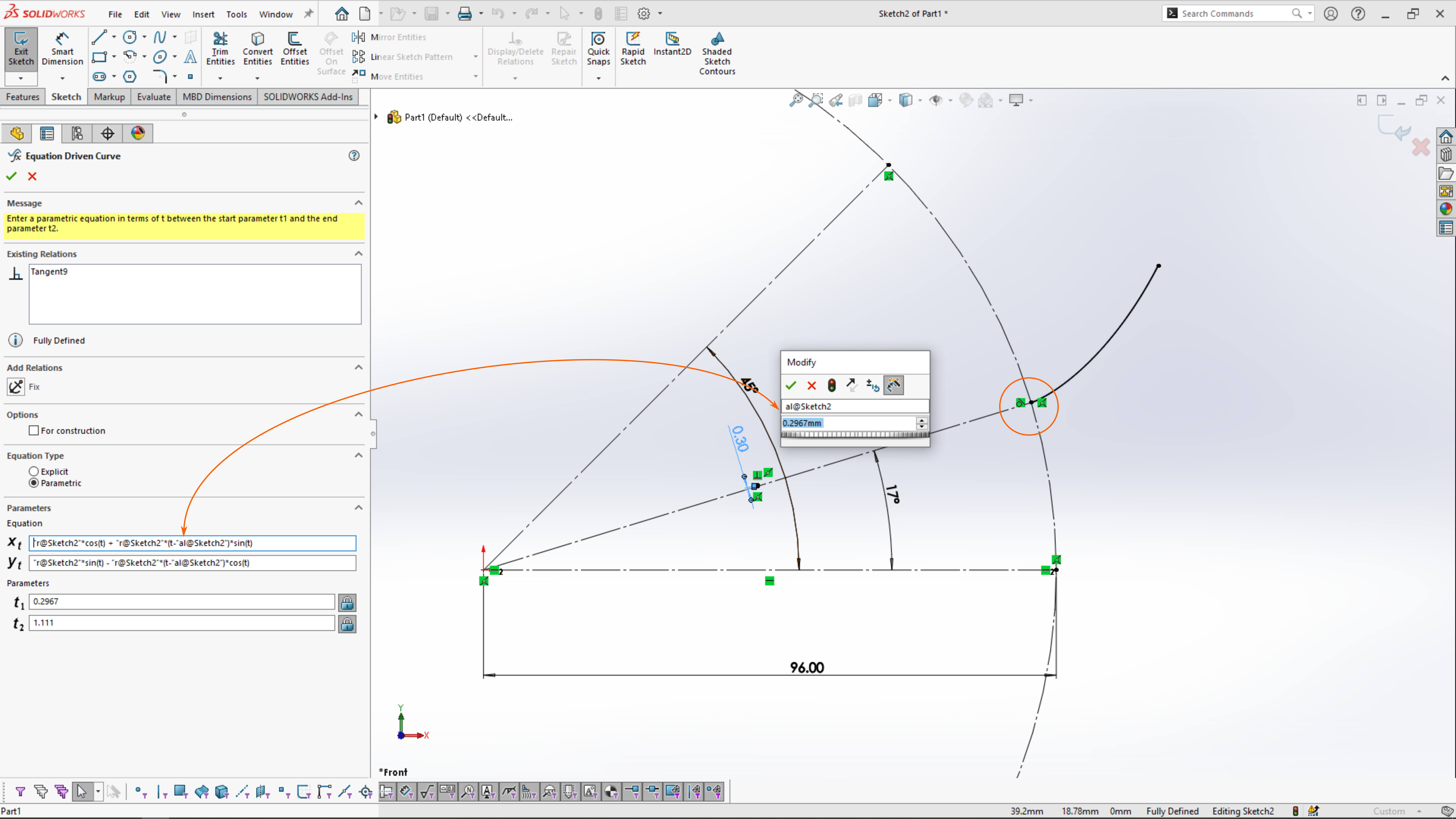The solid line of an involute rooted at α=17∘\alpha = 17^\circα=17∘ leads off the circle, following the parametric curve xtx_txt​, yty_tyt​ between t1t_1t1​ and t2t_2t2​. Giving a construction line the length of 'al' in radians avoids a unit error in the equation curve, an intermediary step that enables part properties to drive the curve. The curve specified by xtx_txt​, yty_tyt​ is not fixed to the sketch origin, requiring coincident and tangent constraints.

The preceding figures of involutes were exaggerated to better show any geometry or plotting errors; more reasonable is a 32 tooth module 2 gear like this one from SDP-SI. For a metric gear described by module, number of teeth, and pressure angle, we can calculate the equation curve in Julia by:

using Rootsfunction calcThetaHeight(; r, gm, al, ht)  htal(th) = r * ( cos(th-gm) + (th-al)*sin(th-gm) - 1 )  fal(th) = ht - htal(th) #the objective function  return find_zero(fal, gm + (gm-al)*2)endfunction printEquationCurve( gearModule, nTeeth, gamma=π/2, pressureAngle=deg2rad(20) )  addendum = gearModule  dedendum = 1.25*gearModule  pitchD = gearModule * nTeeth  r = (pitchD - 2*dedendum)/2  toothThick = gearModule * pi/2  delta = 2*asin( toothThick / 2/r)  alpha = gamma - delta/2  toothHeight = addendum + dedendum  # p = InvoluteTooth.plotTooth( r=r, gm=gamma, dl=delta, ht=toothHeight )  # display(p)  thal = calcThetaHeight(r=r, gm=gamma, al=alpha, ht=toothHeight) #the find_zero function  println("Equation Curve with r=$r and δ=$delta for a $nTeeth module$gearModule gear: ")  println("xt = $r*cos(t) +$r*(t-$alpha)*sin(t)") println("yt =$r*sin(t) - $r*(t-$alpha)*cos(t)")  println("t1 = $alpha") println("t2 =$thal")end

Equation Curve with r=29.5 and δ=0.1065 for a 32 module 2 gear:
xt = 29.5 * cos(t) + 29.5 * (t-1.5175) * sin(t)
yt = 29.5 * sin(t) - 29.5 * (t-1.5175) * cos(t)
t1 = 1.5175
t2 = 2.0905

Excepting Solidworks' curve approximations, the resulting half-tooth, blue, is close to the manufacturer's model through the flank. They appear to use a smaller tooth height than calculated by the standard, but gearing is full of optimizations. The many textbook-quality descriptions of gearing are enough to produce a basic gear, but greater accuracy requires consulting industry standards. While the comparison to the manufacturer's model is favorable, the model should only be treated as an approximate representation of the real part.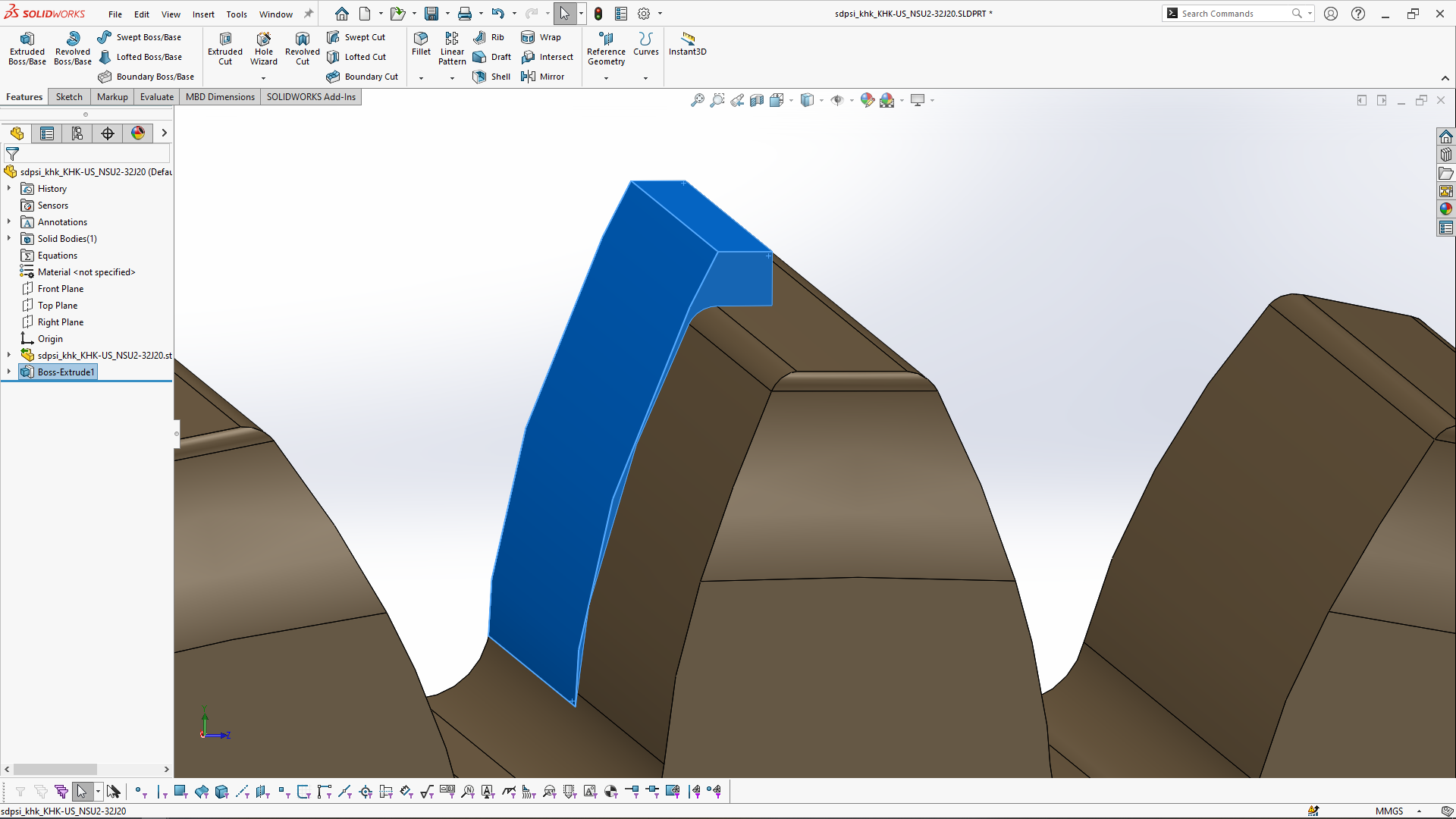The rear half of the tooth, in blue, was calculated and drawn from the equation curve output above. Ignoring Solidworks' curve approximation artifacts, the flanks match well while the calculated tooth height exceeds that of the manufacturer's model.

## Wrapping up #

Despite the many references that discuss gearing, all that I have seen gloss over the drawing of the tooth involutes by computer or in CAD. This presentation was brief but linked the basic equation of an involute to its use in generating gear teeth. The plots here were generated by my InvoluteTooth module and plotted via Plots.jl. Real gears have many additional features that were not modeled here, but I hope this post provides a quick starting point to drawing involute gears. If this left you wanting a more thorough treatment, reach out so that I know what to expand.

As always, comment on Twitter or LinkedIn, and subscribe to see next month's mechanism.